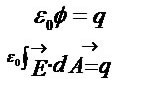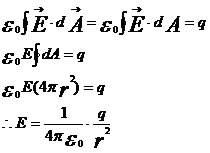Electricity & Megnetism

# Gauss law

Gauss’s law relates electric fields at points on a closed surface to the net charge that is enveloped by that surface.

Gauss’s law relates the resulting electric flux Φ of an electric field, through a closed surface, to the resultant charge that is enclosed by that surface. In other words, Gauss’s law relates the electric fields at points on a (closed) Gaussian surface to the net charge enclosed by that surface.
Mathematically, Gauss’ law is represented by the equation:Where:
ε0 = constant of electrical permittivity in vacuum
Φ = resultant electrical flux
q = electrical charge involved

In the equation, “q” is the algebraic sum of all charges involved, whether positive or negative. It is important to note that the sign says something about the resulting flow. If q is greater than zero, the resulting flow is outward; if q is less than zero, the resulting flux is inward.

A charge outside the Gaussian surface, no matter its size or proximity, is not included in the q term of Gauss’ law. Nor does it matter the exact shape or location of the charges within the Gaussian surface, only the sign of the resulting charge involved matters.
The electric field, due to a charge outside the Gaussian surface, does not contribute any resultant flux through the surface, because the number of field lines, due to this charge entering the surface, is the same as that leaving it.
We can say that Gauss’s law is equivalent to Coulomb’s Law, because we can deduce Coulomb’s law from Gauss’s law.This equation is exactly the equation of the electric field, deduced through the Gauss equation.